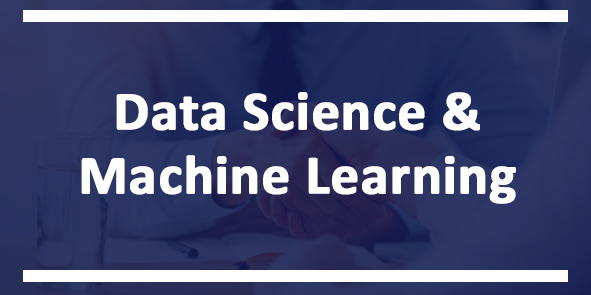# Data Science & Machine Learning Course

## Learn from experts about the fastest-growing job requirement in the industry!

Launch Offer!Click on Details for Syllabus

An expert trainer will help you learn Data Science with Machine Learning!

Features of the course:

1) A detailed day-wise schedule will be provided to get maximum results.

2) Certification after completion of course

3) Create industry-ready projects at the end of course.

4) You can watch courses at any time on a laptop as well as mobile. It's a recorded course.

5) The course has been recorded as an interaction between trainer and one demo student to make it interactive.

Click on the details to check the syllabus.

Projects Included in Course:

1. Bank Loan interest rate prediction model

2. Income classification model

3. Spam detection classification model

4. Crude or money text classification model

 Complete Curriculum of Data Science & Machine Learning
 1. Applications of Machine Learning 2. Why Machine Learning is the Future 3. Update: Recommended Anaconda Version
 Part 1: Data Preprocessing
 4. Welcome to Part 1 - Data Preprocessing 5. Get the dataset 6. Importing the Libraries 7. Importing the Dataset 8. For Python learners, summary of Object-oriented programming: classes & objects 9. Missing Data 10. Categorical Data 11. Splitting the Dataset into the Training set and Test set 12. Feature Scaling 13. And here is our Data Preprocessing Template!
 Statistics
 14. Mean, Median, Mode 15. Standard Deviation, Variance 16. Quartile, Range 17. Central Limit Theorem 18. Correlation 19. Covariance 20. Mean Square Error 21. Probability Distributions Part 2: Regression 22. Welcome to Part 2 - Regression Simple Linear Regression 23. How to get the dataset 24. Dataset + Business Problem Description 25. Simple Linear Regression Intuition 26. Simple Linear Regression in Python Quiz 2: Simple Linear Regression Decision Tree Regression 27. Decision Tree Regression Intuition 28. How to get the dataset 29. Decision Tree Regression in Python Random Forest Regression 30. Random Forest Regression Intuition 31. How to get the dataset 32. Random Forest Regression in Python Evaluating Regression Models Performance 33. R-Squared Intuition 34. Evaluating Regression Models Performance 35. Interpreting Linear Regression Coefficients 36. Conclusion of Part 2 - Regression Part 3: Classification 37. Welcome to Part 3 - Classification Logistic Regression 38. Logistic Regression Intuition 39. How to get the dataset 40. Logistic Regression in Python 41. Python Classification Template Quiz 4: Logistic Regression Evaluating Classification Models Performance 42. False Positives & False Negatives 43. Confusion Matrix 44. Accuracy Paradox 45. CAP Curve 46. CAP Curve Analysis 47. Conclusion of Part 3 - Classification Decision Tree Classification 48. Decision Tree Classification Intuition 49. How to get the dataset 50. Decision Tree Classification in Python Random Forest Classification 51. Random Forest Classification Intuition 52. How to get the dataset 53. Random Forest Classification in Python Part 4: Model Selection & Boosting 54. Welcome to Part 10 - Model Selection & Boosting Model Selection 55. How to get the dataset 56. k-Fold Cross Validation in Python 57 Grid Search in Python 58. Random Search in Python XGBoost 59. How to get the dataset 60 XGBoost in Python K-Nearest Neighbors (K-NN) 61 K-Nearest Neighbor Intuition 62. How to get the dataset 63. K-NN in Python Quiz 5: K-Nearest Neighbor Support Vector Machine (SVM) 64. SVM Intuition 65. How to get the dataset 66. SVM in Python Naive Bayes 67. Bayes Theorem 68. Naive Bayes Intuition 69. How to get the dataset 70 Naive Bayes in Python Part 5: Clustering 71. Welcome to Part 4 - Clustering K-Means Clustering 72. K-Means Clustering Intuition 73. K-Means Random Initialization Trap 74. K-Means Selecting The Number Of Clusters 75. How to get the dataset 76. K-Means Clustering in Python Quiz 6: K-Means Clustering Hierarchical Clustering 77. Hierarchical Clustering Intuition 78. Hierarchical Clustering How Dendrograms Work 79. Hierarchical Clustering Using Dendrograms 80. How to get the dataset 81. HC in Python Quiz 7: Hierarchical Clustering 82. Conclusion of Part 4 - Clustering₹4999 ₹2499

### This package includes :

CoursesData Science with Machine Learning

Learn from experts about the fastest-growing job requirement in the industry!